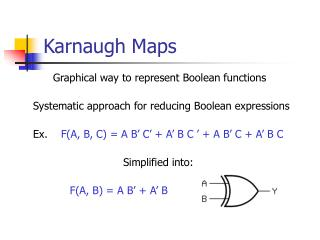DownloadDownload PresentationKarnaugh Maps

# Karnaugh Maps

Download Presentation## Karnaugh Maps

- - - - - - - - - - - - - - - - - - - - - - - - - - - E N D - - - - - - - - - - - - - - - - - - - - - - - - - - -
##### Presentation Transcript

1. Karnaugh Maps Graphical way to represent Boolean functions Systematic approach for reducing Boolean expressions Ex. F(A, B, C) = A B’ C’ + A’ B C ’ + A B’ C + A’ B C Simplified into: F(A, B) = A B’ + A’ B

2. Definition of Kmap • Kmap is a table used to enumerate the values of a given Boolean expression for different input values. • The ROWS and columns of the table correspond to the possible VALUES of the function’s inputs. • Each CELL represents the OUTPUTSof the function for the possible inputs.

3. Minterms in Kmaps • Minterm represents ALL of the possible INPUT combinations for the function. • A function with n variables has 2^n minterms (since each variable can appear complemented or not)

4. Example 1.Re-arranging the truth table into the Kmap for two variables.F(X, Y) = X + YThis function is equivalent to the OR of all of the minterms that have a value of 1. Thus: F(X, Y) = X + Y =X’ Y + X Y’ + X Y X 0 1 Y 0 1

5. The rules of Kmap simplification are: • Groupings can contain only 1s; no 0s. • Groups can be formed only at right angles; diagonal groups are not allowed. • The number of 1s in a group must be a power of 2 – even if it contains a single 1. • The groups must be made as large as possible. • Groups can overlap and wrap around the sides of the Kmap.

6. Example 1. Kmapsimplification for two variablesF(X, Y) = X + Y X 0 1 Y 0 1 • We can begin with the group represented by the second row (where x = 1) • The two minterms are X Y’ and X Y • This group presents the logical OR of these two terms, or X Y’ + X Y. These terms differ in Y, so Y is discarded leaving only X • Thesecond group represents X’ Y and X Y ( X’ Y + X Y differ in X, so X is discarded, leaving Y) • Coming back to our Boolean function: F(X, Y) = X + Y =X’ Y + X Y’ + X Y = X + Y

7. Kmap simplification for three variables • We have placed each minterm in the cell that will hold its value.

8. Example 2. Kmap simplification for three variablesF(X, Y, Z) = X’ Y’ Z + X’ Y Z + X Y’ Z + X Y Z Kmap is given below has the largest group of 1’s that is power of 2. This grouping tells us that changes in the variables X and Y have no influence upon the value of the function: They are irrelevant. • This means that the function, reduces to F(Z) = Z.

9. Example 3. Kmap simplification for three variables.F(X, Y, Z) = X’ Y’ Z’ + X’ Y’ Z + X’ Y Z + X’ Y Z’ + X Y’ Z’ + X Y Z’ • Example of a group that wraps around the sides of a Kmap. • This group tells us that the values of xand y are not relevant to the term of the function that is encompassed by the group leaving only Z’ • The green group in the top row tells us that only the value of x is significant in that group. • X complemented in that row, so the other term of the reduced function is X’ • The reduced function isF(X, Z) = Z’ + X’

10. Example 4. Kmap for four variables • Number of minterms is 2^n, where n=number of variables. In this case we have 2^4 or 16 minterms. Recall that groups can overlap.

11. Possible groupings • Try to keep groups as large as possible. • We may have a choice as to how to pick groups within Kmap, while keeping the groups as large as possible. • The (different) functions that result from the groupings below are logically equivalent.